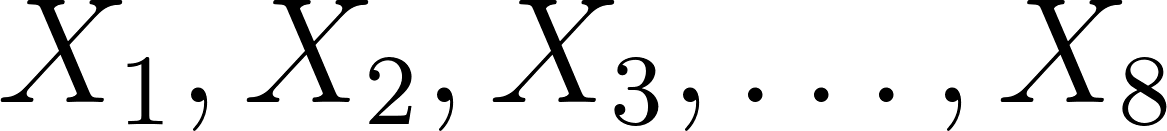×
Get Full Access to Probability And Statistical Inference - 9 Edition - Chapter 5.3 - Problem 19e
Get Full Access to Probability And Statistical Inference - 9 Edition - Chapter 5.3 - Problem 19e

×

# Flip n = 8 fair coins and remove all that came up heads.ISBN: 9780321923271 41

## Solution for problem 19E Chapter 5.3

Probability and Statistical Inference | 9th Edition

• Textbook Solutions
• 2901 Step-by-step solutions solved by professors and subject experts
• Get 24/7 help from StudySoup virtual teaching assistantsProbability and Statistical Inference | 9th Edition

4 5 1 248 Reviews
30
3
Problem 19E

Problem 19E

Flip n = 8 fair coins and remove all that came up heads. Flip the remaining coins (that came up tails) and remove the heads again. Continue flipping the remaining coins until each has come up heads. We shall find the pmf of Y, the number of trials needed. Let Xi equal the number of flips required to observe heads on coin

i, i = 1, 2, . . . , 8. Then Y = max(X1,X2, . . . ,X8).

(a) Show that P(Y y) = [1 − (1/2)y]8.

(b) Show that the pmf of Y is defined by P(Y = y) =[1 − (1/2)y]8 − [1 − (1/2)y−1]8, y = 1, 2, . . . .

(c) Use a computer algebra system such as Maple or Mathematica to show that the mean of Y is E(Y) =13,315,424/3,011,805 = 4.421.

(d) What happens to the expected value of Y as the number of coins is doubled?

Step-by-Step Solution:

Step 1 of 8

We assume that the coin tossesare independent.

We have one chance out of 2 to toss heads:Step 2 of 8

Step 3 of 8

##### ISBN: 9780321923271

Unlock Textbook Solution